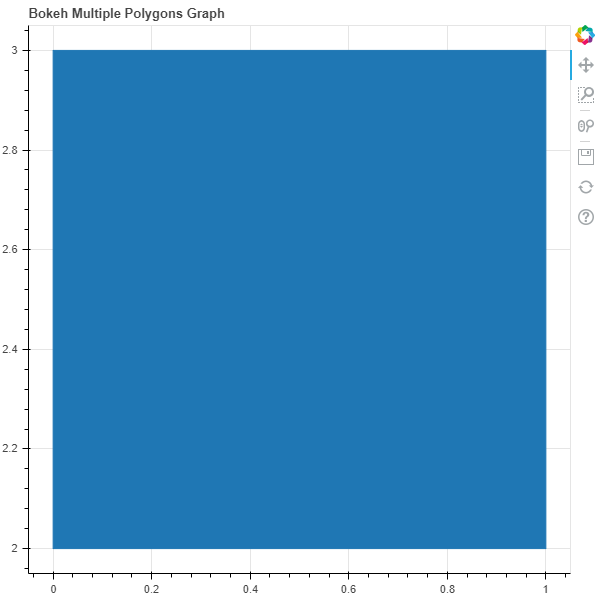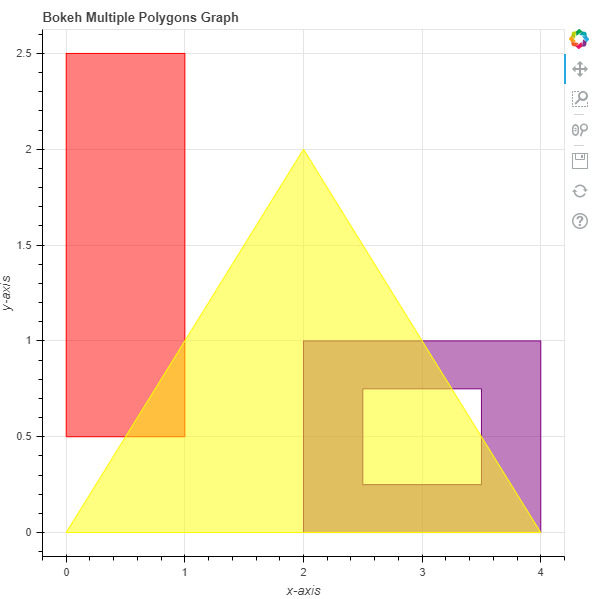# Python Bokeh – Plotting Multiple Polygons on a Graph

Bokeh is a Python interactive data visualization. It renders its plots using HTML and JavaScript. It targets modern web browsers for presentation providing elegant, concise construction of novel graphics with high-performance interactivity.
Bokeh can be used to plot multiple polygons on a graph. Plotting multiple polygons on a graph can be done using the multi_polygons() method of the plotting module.

## plotting.figure.multi_polygons()

Syntax : multi_polygons(parameters)
Parameters :

• xs : x-coordinates of the polygons
• ys : y-coordinates of the polygons
• fill_alpha : fill alpha values of the polygons
• fill_color : fill color values of the polygons
• hatch_alpha : hatch alpha values of the polygons, default is 1
• hatch_color : hatch color values of the polygons, default is black
• hatch_extra : hatch extra values of the polygons
• hatch_pattern : hatch pattern values of the polygons
• hatch_scale : hatch scale values of the polygons, default is 12
• hatch_weight : hatch weight values of the polygons, default is 1
• line_alpha : percentage value of line alpha, default is 1
• line_cap : value of line cap for the line, default is butt
• line_color : color of the line, default is black
• line_dash : value of line dash such as : solid, dashed, dotted, dotdash, dashdot [default is solid ]
• line_dash_offset : value of line dash offset, default is 0
• line_join : value of line join, default in bevel
• line_width : value of the width of the line, default is 1
• name : user-supplied name for the model
• tags : user-supplied values for the model

Other Parameters :

• alpha : sets all alpha keyword arguments at once
• color : sets all color keyword arguments at once
• legend_field : name of a column in the data source that should be used
• legend_group : name of a column in the data source that should be used
• legend_label : labels the legend entry
• muted : determines whether the glyph should be rendered as muted or not, default is False
• name : optional user-supplied name to attach to the renderer
• source : user-supplied data source
• view : view for filtering the data source
• visible : determines whether the glyph should be rendered or not, default is True
• x_range_name : name of an extra range to use for mapping x-coordinates
• y_range_name : name of an extra range to use for mapping y-coordinates
• level : specifies the render level order for this glyph

Returns : an object of class GlyphRenderer

Example 1 : In this example we will be using the default values for plotting the graph.

## Python3

 `# importing the modules ` `from` `bokeh.plotting ``import` `figure, output_file, show `   `# file to save the model ` `output_file("gfg.html") ` `     `  `# instantiating the figure object ` `graph ``=` `figure(title ``=` `"Bokeh Multiple Polygons Graph") ` `   `  `# the points to be plotted` `xs ``=` `[[[[``0``, ``0``, ``1``, ``1``]]]]` `ys ``=` `[[[[``3``, ``2``, ``2``, ``3``]]]]` `    `  `# plotting the graph ` `graph.multi_polygons(xs, ys) ` `     `  `# displaying the model ` `show(graph)`

Output :Example 2 : In this example we will be plotting the multiple polygons with various other parameters

## Python3

 `# importing the modules ` `from` `bokeh.plotting ``import` `figure, output_file, show `   `# file to save the model ` `output_file("gfg.html") ` `     `  `# instantiating the figure object ` `graph ``=` `figure(title ``=` `"Bokeh Multiple Polygons Graph") `   `# name of the x-axis ` `graph.xaxis.axis_label ``=` `"x``-``axis"` `     `  `# name of the y-axis ` `graph.yaxis.axis_label ``=` `"y``-``axis"`   `# the points to be plotted` `xs ``=` `[[[[``0``, ``0``, ``1``, ``1``]]],` `      ``[[[``2``, ``2``, ``4``, ``4``], [``2.5``, ``2.5``, ``3.5``, ``3.5``]]],` `      ``[[[``2``, ``0``, ``4``]]]]` `ys ``=` `[[[[``2.5``, ``0.5``, ``0.5``, ``2.5``]]],` `      ``[[[``1``, ``0``, ``0``, ``1``], [``0.75``, ``0.25``, ``0.25``, ``0.75``]]],` `      ``[[[``2``, ``0``, ``0``]]]]`   `# color values of the polygons` `color ``=` `["red", "purple", "yellow"]`   `# fill alpha values of the polygons` `fill_alpha ``=` `0.5`   `# plotting the graph ` `graph.multi_polygons(xs, ys,` `                     ``color ``=` `color,` `                     ``fill_alpha ``=` `fill_alpha) ` `     `  `# displaying the model ` `show(graph)`

Output :Whether you're preparing for your first job interview or aiming to upskill in this ever-evolving tech landscape, GeeksforGeeks Courses are your key to success. We provide top-quality content at affordable prices, all geared towards accelerating your growth in a time-bound manner. Join the millions we've already empowered, and we're here to do the same for you. Don't miss out - check it out now!

Previous
Next